# 2.7. MEMORY HIERARCHY AND PARALLEL PROGRAMMING TOOLS

## 2.6. PARALLEL LANGUAGES

C and Fortran 77 cannot be used the same way for vector and superscalar processors as they are for serial scalar ones. These languages do not reflect certain essential features of these architectures, and thus make the writing of efficiently portable programs for vector and superscalar processors difficult or even impossible.

Optimizing compilers can only solve this problem for a simple and limited class of applications. Array libraries are able to provide efficient implementation for a relatively small number of array operations, not covering all possible operations on arrays that may be needed for an algorithm. More complex array operations can only be expressed as a combination of the locally optimized library array operations. This approach excludes global optimization of combined array operations and therefore can only provide a relatively slow implementation for many algorithms, potentially suitable for a much more efficient execution on vector and superscalar processors.

Parallel extensions of C and Fortran 77, which allow the programmer to explicitly express in a portable way array-based computations that can be efficiently implemented for any vector and superscalar processor, provide a comprehensive solution to the problem of efficiently portable programming of these architectures. The compiler for a parallel language does not recognize what portions of source code are suitable for efficient execution on vector and superscalar processors. The compiler just focuses on the generation of efficient target code for the array operations specified by the programmer. In generating the target code, the compiler is potentially able to optimize the array-based computations as global as it is allowed by the modular structure of source code.

A number of parallel supersets of C and Fortran 77 have been designed for efficiently portable programming vector and superscalar processors. In the book, we outline two of them: Fortran 90 and C[ ].

### 2.6.1. Fortran 90

Fortran 90 is a new standard of the Fortran language released in 1991. It has been widely implemented in recent years. Fortran 90 adds many powerful extensions to the Fortran language. The new features of Fortran 90 can be divided into two categories based on their motivation. The first category unites the features aimed at modernization of the language according to the state-of-the-art in serial programming languages in order to make Fortran competitive with computer languages created later (e.g., C and C+). The features of the category include

• free-format source code and some other simple improvements;

• dynamic memory allocation (automatic arrays, allocatable arrays, and pointers and associated heap storage management);

• user-defined data types (structures);

• generic user-defined procedures (functions and subroutines) and operators;

• recursive procedures;

• new control structures to support structured programming;

• a new program unit, MODULE, for encapsulation of data and a related set of procedures.

These extensions upgrade the archaic syntax of Fortran 77 and introduce modern storage management, a control structure, a procedural structure, and a basic support for object-oriented programming. The new features are very important for Fortran programmers, but they are not directly related to parallel programming vector and superscalar architectures.

The second category of added features includes the extensions made to support explicit expression of operations on arrays. Unlike Fortran 77, Fortran 90 considers arrays first-class objects and allows whole-array operations, assignments, and functions. In the case of arrays, operations and assignments are extended in an obvious way—element by element. So, for the arrays

` REAL, DIMENSION(3,4,5) :: a, b, c`

the statement

`c = a + b`

performs elementwise addition of the arrays a and b, storing the result in the array c.

Similarly all appropriate intrinsic functions are array-valued for array arguments. They operate elementwise if given an array as their argument. Therefore the statement

`c = SQRT(a)`

is legal in Fortran 90 and has the obvious semantics. Fortran 90 also allows the programmers to write array-valued functions themselves.

Array expressions may also include scalar constants and variables, which are replicated (or expanded) to the required number of elements. For example, the statement

`c = a + 2.0`

increases all elements of the array a by 2.0, storing the result in the array c.

Occasionally some elements of arrays in an array-valued expression must be treated specially. This is done by using the WHERE structure. For example, to avoid division by zero in the statement

`a = 1./a`

where a is an array, the WHERE statement

` WHERE (a / = 0.) a = 1./a`

or the WHERE construct

` WHERE (a / = 0.)       a = 1./a ELSEWHERE       a = HUGE(a) END WHERE `

can be used.

All the array elements in an array-valued expression or array assignment must be conformable. That means that they must have the same shape, that is, the same number of axes as well as the same number of elements along each axis. For example, all the arrays a, b, and c

` REAL :: a(3,4,5), b(0:2,4,5), c(3,4,-1:3)`

have the same rank of 3, extents of 3, 4, and 5, shape of {3, 4, 5}, and size of 60. They only differ in the lower and upper dimension bounds. The first dimension of the array b ranges from 0 to 2, while that of the arrays a and c ranges from 1 to 3. The third dimension of the array c ranges from -1 to 3, while that of the arrays a and b ranges from 1 to 5. Thus the statement

`c = b/SQRT(ABS(a)+1.)`

is correct because all of its array elements are conformable.

Whole arrays are not the only array-valued objects allowed in Fortran 90. An array section can be used everywhere in array assignments and array-valued expressions where a whole array is allowed. In general, an array section is specified with triplets of the form

`lower : upper : stride`

used as subscripts. The triplet designates an ordered set of integer values i1,…, ik such that i1 = lower and ij+1 = ij + stride (j = 1,…, k - 1), and | ik - upper | < stride. Thus for the array

` REAL :: a(50,50)`

the expression

`a(i,1:50:1) or a(i,1:50) or a(i,:)`

designates the ith row of array a. This rank 1 section has a shape of {50}. Note that the third component of the triplet expression along with the

preceding semicolon may be omitted. In that case the stride is set to 1. The first and/or the second components may also be omitted. In that case the lower and/or upper bounds will be taken from the array’s declaration. The expression

`a(i,1:50:3)`

selects every third element of this row. The shape of this section is {16}. The expression

`a(i,50:1:-1)`

designates this row in reverse order. The section is also of rank 1 and has a shape of {50}. The expression

`a(11:40,j)`

designates a part of the jth column (a section of the shape {30}). The expression

`a(1:10,1:10)`

designates the left upper 10 X 10 block of a, which is a rank 2 section of the shape {10, 10}.

Along with triplets, vector subscripts may also be used to specify array sections. Any expression whose value is a rank 1 integer array may be used as a vector subsript. For example, the code

` REAL :: a(5,5), b(5) INTEGER :: index(5) index = (/5,4,3,2,1/) b = a(index,1)`

assigns the reversed first column of array a to array b. The array-valued variable index is used as a vector subscript in expression a(index,1) designating an array section.

Whole arrays and array sections of the same shape can be mixed in expressions and assignments. Note that unlike a whole array, an array section may not occupy contiguous storage locations.

Along with array variables and array-valued expressions, Fortran 90 introduces array constants, which is quite natural as soon as arrays are considered first-class objects. Array constants may appear in any array expression. The simplest form of array constant is just a list of elements enclosed in (/ and /). Such an array constant was used in the example above to initialize the array index. In general, array constants, also known as array constructors, may contain lists of scalars, lists of arrays, and implied-DO loops familiar from I/O lists. Some examples of correct array constants are

`(/ 0, i=1,50 /) (/ (3.14*i, i=4,100,3) /) (/ ( (/ 5,4,3,2,1 /), i=1,5 ) /)`

The array constructors are only able to produce one-dimensional arrays. In order to construct arrays of higher rank, the function RESHAPE can be used. The second argument of the function is the shape of the output array, while its first argument specifies the array whose elements should be used for constructing the output array of the specified shape. For example, in order to assign zeros to all elements of the array a,

` REAL :: a(500,500)`

the statement

`a = RESHAPE( (/ (0., i=1,250000) /), (/ 500,500 /) )`

can be used.

User-defined procedures implementing operations on arrays normally use assumed-shape arrays as formal array arguments. For example, the subprogram

` SUBROUTINE swap(a,b) REAL, DIMENSION(:,:) :: a, b REAL, DIMENSION(SIZE(a,1), SIZE(a,2)) :: temp temp = a a = b b = temp END SUBROUTINE swap`

has two formal array arguments a and b, whose specification only defines their type and rank, but bounds are just marked with colons. This means that the actual shape is taken from that of the array arguments each time the subroutine is called. The local array temp is an example of the automatic array. Its size is set at runtime with the intrinsic function SIZE, which returns the number of elements in the dimension, specified by the second argument, of the array specified by the first argument. The automatic array are released as soon as control leaves the procedure in which it is defined.

In addition to extension of such intrinsic functions as SQRT and SIN to array arguments, Fortran 90 introduces a number of specific array intrinsic functions. The functions do the following:

• Compute the scalar product of two vectors (the function DOT_PRODUCT) and the matrix product of two matrices (the function MATMUL).

• Perform diverse reduction operations on an array, such as logical multiplication (the function ALL) and addition (the function ANY) of the elements of the array, counting the number of true elements in the array, arithmetical multiplication (the function PRODUCT) and addition (the function SUM) of its elements, and finding the smallest (the function MINVAL) or the largest (the function MAXVAL) element of the array.

• Return diverse attributes of an array such as its shape (the function SHAPE), the lower-dimension bounds of the array (the function LBOUND), the upper-dimension bounds (the function UBOUND), the number of elements (the function SIZE), and the allocation status of the array (the function ALLOCATED).

• Construct arrays by means of merging two arrays under mask (the function MERGE), or packing an array into a vector (the function PACK), or replication of an array by adding a dimension (the function SPREAD), or unpacking a vector (a rank 1 array) into an array under mask (the function UNPACK).

• Reshape arrays (the function RESHAPE).

• Move array elements performing the circular shift (the function CSHIFT), or the end-off shift (the function EOSHIFT), or the transpose of a rank 2 array (the function TRANSPOSE).

• Locate the first maximum (the function MAXLOC) or minimum (the function MINLOC) element in an array.

### 2.6.2. The C[ ] Language

The C[ ] (C “brackets”) language is a strict ANSI C superset allowing programmers to explicitly describe operations on arrays.

2.6.2.1. Vector Value and Vector Object. A basic new notion of the C[ ] language is vector value, or simply vector. Vector value is an ordered sequence of values (or vector values) of any one type. Any vector type is characterized by two attributes: the number of elements and the type of elements.

ANSI C defines object as the region of data storage whose contents can represent values. The C[ ] language introduces the notion of vector object as the region of data storage whose contents can represent vector values. Namely C[ ] defines vector object as an ordered sequence of objects (or vector objects) of any one type.

ANSI C does not define the notion of value of array object. The C[ ] language does, and this value is a vector. For example, the value of the array defined with the declaration

` int a = {0,1,2,3,4,5}; `

is the vector

`{ {0,1}, {2,3}, {4,5} }`

consisting of three vectors, each composed of two integers. This vector type is named by the type name int_. We also say that the vector has the shape {3, 2}. By definition, the shape of the array is the same as that of its vector value. Thus, in C[], array object is a particular case of vector object.

2.6.2.2. Arrays and Pointers. In C, an array comprises a contiguously allocated set of elements of any one type of object. In C[ ], an array comprises a set of elements of any one type of object sequentially allocated with a positive stride. The stride is a distance between successive elements of the array measured in units equal to the size of array element. If stride is not specified, it is assumed to be 1.

Thus a C[ ] array has at least three attributes: the type of elements, the number of elements, and the allocation stride. For example, both the declaration

` int a;`

and the declaration

` int a[3:1];`

define an array of the formThe declaration

` int a[3:3];`

defines an array of the formThe size of the slot between array elements is 2 X sizeof (int) bytes.

In C, a pointer has only one attribute—the type of object it points to. This attribute is necessary for the correct interpretation of the value of the object

it points to as well as the address operators + and -. These operators are correct only if the pointer’s operands and the pointer’s results point to elements of the same array object. The same rule is valid for the C[ ] language. Therefore, to support the correct interpretation of the address operators, C[ ] introduces one more attribute of the pointer; this attribute is stride. If stride is not specified, it is equal to 1.

In C, when an expression that has integer type is added to or subtracted from a pointer, the integer value is first multiplied by the size of the object pointed to. In C[ ], the multiplier is equal to the product of the pointer’s stride and the size of the object pointed to. In C, when two pointers to elements of the same array object are subtracted, the difference is divided by the size of an element. In C[ ], the divisor is equal to the product of the pointer’s stride and the size of an element.

For example, the declarations

` int  _  a[] = {0,1,2,3,4}; int *  _p1  = (void*)a; int *:2 p2  = (void*)&a;`

form the following structure of storage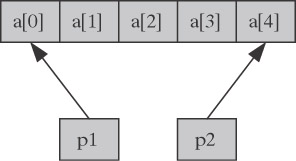The address expressions p1+2 and p2-1 point to the same element of the array a, namely a. Indeed, the offset from p1 specified by the expression p1+2 is equal to (1 X sizeof (int)) X 2 = 2 X sizeof (int) bytes, and the offset from p2 specified by the expression p2-1 is equal to (2 X sizeof (int)) X (-1) = -2 X sizeof (int) bytes.

In C[ ], access to the e2-th element of an array object e1 is obtained with using one of the expressions e1[e2] or (e2)[e1]. Both are identical to (*(((e1)+(e2)))). Here, e2 is an integer expression, e1 is an lvalue that has the type “array of type.” This lvalue is converted to an expression that has the type “pointer to type” and that points to the intial element of the array object (the attribute stride of the pointer is identical to that of the array object).

In C[ ], an integer nonconstant expression may be used to specify the size of an automatic array, or the stride of an atomatic array or pointer. The following fragment of C[ ] code, which initializes with units the diagonal elements of an n X n matrix, illustrates the use of such dynamic arrays and pointers:

` typedef int (*pDiag)[n:n+1]; int a[n][n]; int j; pDiag p = (void*)a; ... for(j=0; j<n; j++)       (*p)[j]=1;`

2.6.2.3. Lvector. In C[ ], the value of an array object is a vector. The ith element of the vector is the value of the ith element of the array object.

To support access to an array as the whole, C[ ] introduces the postfix [ ] operator (the blocking operator). The operand of the operator has the type “array of type.” The [ ] operator blocks the conversion of the operand to a pointer. For example, if the arrays a, b, and c are declared as

` int a, b[5:2], c[5:3];`

then expressions a[], b[], and c[] designate arrays a, b, and c as a whole; and expression c[]=a[]+b[] assigns the sum of the vectors that are the values of arrays a and b to the array c. Note that expression a[]+b[] has type “vector of 5 ints.”

In C, an lvalue is an expression designating an object. For example, if the array d is declared as

` int d;`

then expressions d[i][j], d, and d are lvalues, while expressions d[i][j]+1 and &d are not. A modifiable lvalue is an lvalue that is allowed as the left operand of an assignment operator. So, while d[i][j] is a modifiable lvalue, d and d are not.

In addition to lvalue, C[ ] introduces the notion of lvector as an expression designating a vector object. Modifiable lvector is an lvector that can be used as the left operand of an assignment operator. Array object is a particular case of vector object. Therefore expressions d, d, d[], and d[] are lvectors. At the same time, d[] and d[] are modifiable lvectors, meanwhile d and d are not.

In principal, the extended notions of array and pointer in concert with the [ ] operator support the access to whole arrays and subarrays. For example, if array a is declared as

` int a;`

then the modifiable lvector

`(*(int(*)[4:5])a)[] `

designates an array of four ints allocated with stride 5 that contains the main diagonal of matrix a: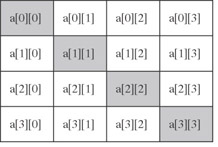We say that an object belongs to an array if it is an element of the array or belongs to an element of the array. By definition, a set of objects belonging to an array is a subarray of the array if and only if the set is an array itself. The main diagonal above is a subarray of array a because it is an array object of the type int[4:5].

The modifiable lvector

`(*(int(*)[3:5])(&a))[]`

designates a subarray of the array a that contains the first superdiagonal of the matrix a: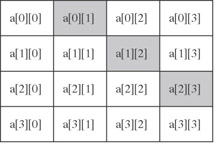Not every, even regular, set of objects belonging to an array is its subarray. For example, if array a is declared as

` int a;`

then the inner elements of matrix a,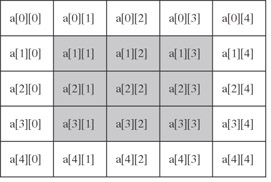do not make up a subarray. Therefore there is no constant modifiable lvector similar to those used for designation of the “diagonal” subarrays that designates this “inner square.”

In order to support access to such array sections of general form, C[ ] introduces the [:] operator (the grid operator). In the general case, this quaternary operator has the following syntax:

` e[l:r:s]`

where expression e may have type “array of type” or “pointer to type” and expressions l, r, and s have integer types and denote the left bound, right bound, and stride respectively. In that case the expression designates a vector object consisting of (r - s)/l + 1 elements of type type. The ith element of the resulting vector object is e[l + s_ _i]. Expression e[l_:_r_:_s] is an lvector. It will be a modifiable lvector, if all the expressions {e[l + s_ _i]}i=0,1,…are either modifiable lvalues or modifiable lvectors. If stride s is equal to 1, the corresponding operand along with the preceding semicolon may be omitted (i.e., e[l_:_r] may be used as a short form of e[l_:_r_:_1]).

The first operand e of the grid operator may have a vector type. In that case the operator is applied elementwise to each element of the vector value of e. For example, let the vector value be {u1,…, uk}. Then the expression e[l_:_r_:_s] will designate a vector of k vectors with the ith element of the jth vector being uj[l + s_ _i] (j = 1,…, k). This successive application of several grid operations allows the programmer to construct modifiable lvectors designating diverse sections of multidimensional arrays (or more precisely, arrays of arrays).

Thus the array section above can be designated with the modifiable lvector a[1:3][1:3]. Indeed, expression a[1:3] is an unmodifiable lvector designating a vector object consisting of three arrays a, a, and a of type int that correspondingly contain the second, third, and fourth rows of the 5 X 5 matrix a: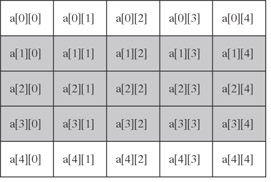This lvector is unmodifiable because a, a, and a are unmodifiable lvalues.

In expression a[1:3][1:3], the second grid operator [1:3] is applied to each of these three arrays selecting their second, third, and forth elements. Therefore expression a[1:3][1:3] designates a vector object consisting of three arrays of the type int that contain the inner 3 X 3 square of the 5 X 5 matrix a: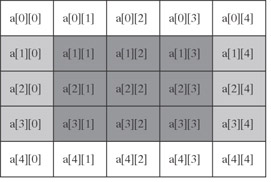The second operand l and/or the third operand r of the grid operator may be omitted. If l is omitted, the left bound is set to 0. If r is omitted, the right bound is set to n - 1 if the first operand e is an n-element array, or determined from the context if e is a pointer. So expression a[:][:] is a modifiable lvector designating as a whole the 5 X 5 array a above. Note that the more compact modifiable lvector a[] also designates this array.

2.6.2.4. Elementwise Vector Operators. The operand of the scalar cast operator and the unary operators &, *, +, -, ~, !, ++, and -may have a vector type. In that case the operators are applied elementwise to each element of the vector operand to produce the vector result.

For example, if array p is defined with declarations

` int j, k, l, m, n; int *p = { &j, &k, &l, &m, &n };`

then expression p[1:3] is a modifiable lvector designating a vector object consisting of three variables p, p, and p of the type int*, and expression *(p[1:3]) is a modifiable lvector designating a vector object consisting of three integer variables k, l, and m.

Any of the binary operators *, /, %, +, -, <<, >>, <, >, <=, ,>=, ==, !=, &, ^, |, &&, and || may have vector operands. If the operands have the same shape, then the operator is executed elementwise to produce the result of this shape.

In general, the operands have different shapes. However, the operands must be conformable in that the beginning of the shape of one operand must be identical to the shape of the other operand. For example, two vectors of shapes {9, 8, 7, 6} and {9, 8} are conformable. A nonvector operand is always conformable with any vector operand because all nonvectors have the empty shape { }. If the rank of one operand a is less than that of the other operand b, then the execution of the operator starts from conformable extension of the vector value of operand a to the shape of operand b. The conformable extension just replicates the vector value by adding dimensions. For example, comformtable extension of the vector

`{ {1,2,3}, {4,5,6} }`

of the shape {2, 3} to the shape {2, 3, 2} is vector

`{ { {1,1}, {2,2}, {3,3} }, { {4,4}, {5,5}, {6,6} } }`

Then the operator is applied elementwise to the result of the conformable extension of the value of operand a and the value of operand b, producing the result of the same shape as that of operand b.

C[ ] introduces two new binary operators, ?> and ?<. The result of the ?> operator is the maximum of its operands. The result of the ?< operator is the minimum of the operands. The operators may also have vector operands. In that case they are executed in the same manner as other binary arithmetic operators.

The assignment operators =, *=, +=, etc., may have vector operands. In that case the left operand of an assignment operator must be a modifiable lvector. Its rank must be not less than the rank of the right operand, and the operands must be conformable. If the rank of the right operand is less than that of the left operand before the elementwise execution of the assignment operator, the value of the right operand is conformably extended to the shape of the left one. For example, the C[ ] code

` int a[m][n], b[m]; ... a[:][:] = b[:];`

assigns the value of the ith element of the array b to all elements of the ith row of the array a (i = 0,…, m - 1). The following is a fragment of the C[ ] application performing the LU factorization of the square n X n matrix a by using the Gaussian elimination:

` double a[n][n], t; int i, j; ... for(i=0; i<n; i++) {       for(j=i+1; j<n; j++) {             t = a[j][i]/a[i][i];             if(a[j][i]!=0.)                   a[j][i:n-1]-=4*a[i][i:n-1];       } }`

The definition of the subcript operator [ ] is that e1[e2] is identical to (*((e1) + (e2))), and the expressions e1 and e2 may be of vector type. This allows the programmer to construct lvectors designating irregular array sections. For example, the C[ ] code

` int a[m][n], ind[] = {0, 1, 6, 18}; ... a[ind[:]][:] = 0;`

zeros the elements of the 0th, 1st, 6th, and 18th rows of array a. The expression a[ind[:]][:] is a modifiable lvector that designates a vector object of shape {4, n} consisting of rows a, a, a, and a of array a.

The first operand of the . operator may have a vector type. The second operand must name a member of a structure or union type. In that case the operator is executed elementwise, and the result will have the same shape as the first operand. Expression e id is identical to expression (*e).id.

2.6.2.5. Reduction operators. For the binary operators *, +, &, ^, |, &&, ||, ?<, and ?>, the C[ ] language introduces reduction operators [*], [+], [&],[^], [|],[&&],[||],[?<], and [?>]. The reduction operators are unary prefix operators only applicable to vector operands. The definition of the reduction operator [ ] is that if v1,…, vn are the elements of the vector memory hierarchy and parallel programming tools

value of expression e, then the value of expression [ ]e is that of expression v1 v2vn. For example, the C[ ] code

` double a[n]; double b[n]; double c; ... c = [+](a[]*b[]);`

computes the dot product of vectors a and b. The code

` int a[m][n]; int max; ... max = [?>][?>]a[];`

computes the maximum element of matrix a. The value of expression a[] is an m-element vector, whose elements are n-element vectors of integers. The value of expression [?>] a[] is an n-element vector whose ith element is the maximum element of the ith column of matrix a. Finally, the application of operator [?>] to this vector yields the maximum of the column maximums, that is, the maximum element of the entire matrix a.

The code

` double a[m][l]; double b[l][n]; double c[m][n]; int i; ... for(i=0; i<m; i++)       c[i][] = [+](a[i][]*b[]);`

multiplies matrices a and b storing the result into matrix c.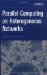Parallel Computing on Heterogeneous Networks (Wiley Series on Parallel and Distributed Computing)
ISBN: B000W7ZQBO
EAN: N/A
Year: 2005
Pages: 95